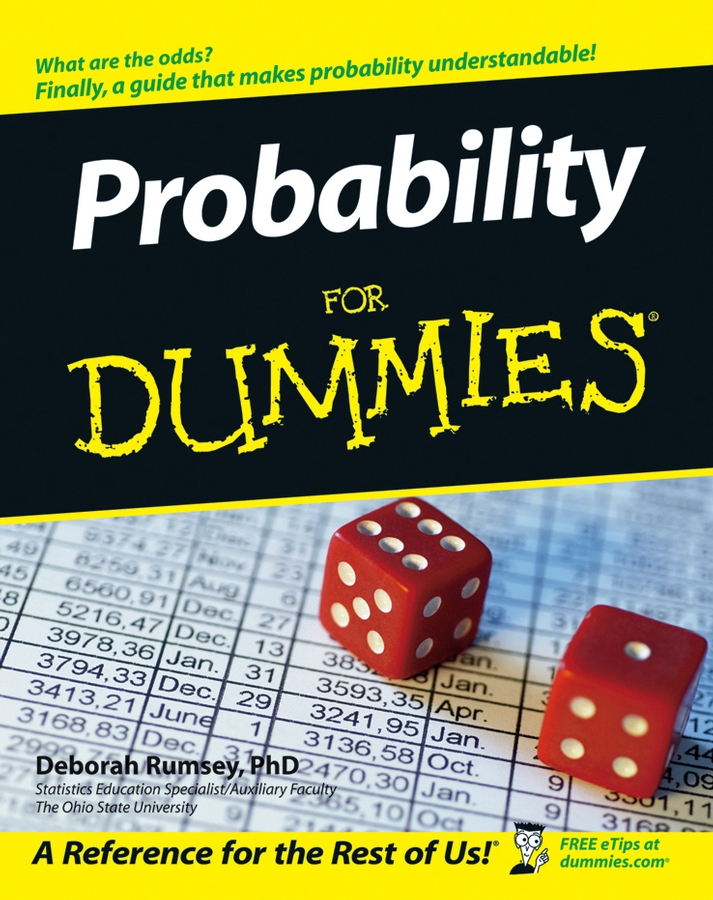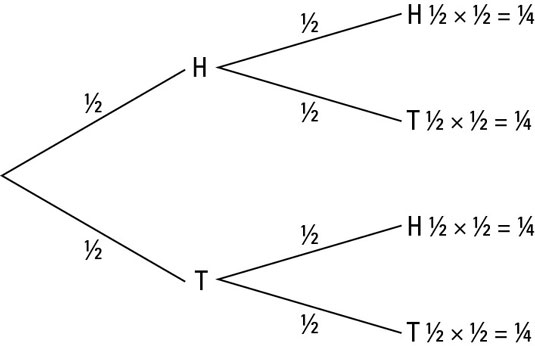##### Probability For DummiesTeachers around the world use probability trees. Probability trees are quite versatile if you use them properly. The idea of a probability tree is to start on the left with ‘the whole thing’ or one. Every time several possible outcomes exist the probability in that branch splits off into a smaller branch for each outcome.

What is the probability of throwing a head and a tail if you toss two coins?At the first event – the first coin toss – you have a 1/2 chance of the coin coming up heads and a 1/2 chance of the coin coming up tails. On the left of the tree, the line splits into two parts: one with H (for heads) at the end, labelled 1/2, and another with T (for tails) at the end, also labelled 1/2.

At the second event – the second coin toss – each of the branches splits again. The outcomes and probabilities are the same as before for each, so you get a branch with H at the end labelled 1/2 and a branch with T at the end, also labelled 1/2.

You can use the tree to work out the probability of any combination of events. For example, to find the probability of the first coin being a head and the second a tail, you follow the branch from the first split to H, and then the branch from there to T, and then times the labels (the probabilities) together. You get a half of a half: 1/2 x 1/2 = 1/4.

Multiplying by a fraction makes your number smaller. As you go across the tree, you split up the probability at each event. Think of a river splitting up into smaller streams as you head towards the source.

Here are the steps for drawing your own probability tree:
1. Decide what happens first and how many possible outcomes there are.

Draw that many branches coming out of a point on the left of the page. Be sure to give yourself plenty of room for labels.

2. For each of the outcomes, work out the probability and write it beside the line.

Also write something at the end of the line to tell you what the outcome is.

3. For each branch, repeat the process.

Count outcomes, draw lines and label.

4. Working from the start point to the end of each branch, times together the probabilities of the branches you go along.

Write the result at the end of the branch.

5. Check that the end probabilities add up to one.

If not, you’ve made a mistake somewhere.

You can find the probability of any combination of events you care about by following the branches through the probability tree.